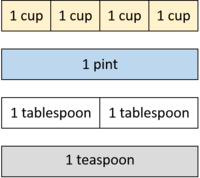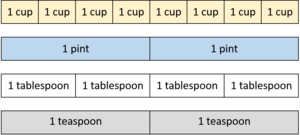##### Engage your students with effective distance learning resources. ACCESS RESOURCES>>

Alignments to Content Standards: 6.RP.A.3

A recipe for bread calls for 4 cups of flour, 1 pint of water, 2 tablespoons of yeast, and 1 teaspoon of salt. The diagram below represents 1 batch of bread.1. How much of each ingredient is needed for 2 batches of bread? Draw a diagram that shows this.
2. How much of each ingredient is needed for 3 batches of bread? Draw a diagram that shows this.
3. How much of each ingredient is needed for 5 batches of bread? (Can you answer this without drawing a diagram?)
4. What is the ratio of flour to yeast in five batches of bread?

## IM Commentary

The primary purpose of this task is to represent ratios of two or more quantities with parallel tape diagrams. Note that the solution to this task assumes that students have already studied equivalent ratios and understand that when you have a context with 8 units of one quantity and 2 units of another quantity, you can say the ratio is 4:1 because it is an equivalent ratio. In general, when people talk about two ratios being the same, they really mean they are equivalent.

Parallel tape diagrams might look a bit strange to the eye at first because, for example, the rectangle representing one teaspoon is larger than the rectangle representing one cup. This is intentional, and engaging in MP.1 to make sense of this diagram is part of the work of this task. In a parallel tape diagram, sets of bars that are the same length represent equivalent ratios. Work with this style of diagram is intended as a stepping stone to students working with and understanding double number line diagrams. (Which are themselves a stepping stone to working with and understanding ratio tables.)

Part (d) opens a door to some class discussion around the fact that 20:10, 10:5, or any ratio equivalent to 2:1 is an acceptable answer. Experienced doers-of-math often default to the smallest possible whole numbers when writing a ratio; however, any number greater than $0$, s, can be used to create a ratio 2s : 1s, and these are all equivalent.

More generally, this task can be used to begin to transition students from an understanding of ratio equivalence in terms of whole-numbers of batches to the more abstract undestanding that $a : b$ is equivalent to any ratio of the form $sa : sb$, where $s$ is any positive value. There are two aspects to this transition. First, students must recognize that when you make $n$ batches of a recipe, you multiply the amount of each of the ingredients by $n$.

Another step of this transition (after this task) will be to scale recipes by non-whole numbers, and to see that to make $1\frac12$ batches, you multiply the amount of each of the ingredients by $1\frac12$. Then students should see that a similar idea applies to other contexts that are not as naturally conceived of in terms of batches, like constant speed and unit pricing.

## Solution

1. Two batches require 8 cups of flour, 2 pints of water, 4 tablespoons of yeast, and 2 teaspoons of salt.2. Three batches require 12 cups of flour, 3 pints of water, 6 tablespoons of yeast, and 3 teaspoons of salt.3. We can multiply the quantity for each ingredient by 5 to get the answer: 20 cups of flour, 5 pints of water, 10 tablespoons of yeast, and 5 teaspoons of salt
4. 20:10 or any equivalent ratio.